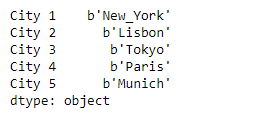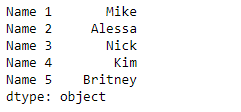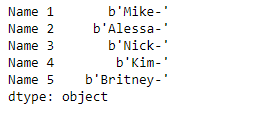Open In App

# Python | Pandas Series.str.encode()

`Series.str` can be used to access the values of the series as strings and apply several methods to it. Pandas` Series.str.encode()` function is used to encode character string in the Series/Index using indicated encoding. Equivalent to `str.encode()`.

Syntax: Series.str.encode(encoding, errors=’strict’)

Parameter :
encoding : str
errors : str, optional

Returns : encoded : Series/Index of objects

Example #1: Use `Series.str.encode()` function to encode the character strings present in the underlying data of the given series object. Use ‘raw_unicode_escape’ for encoding.

 `# importing pandas as pd``import` `pandas as pd`` ` `# Creating the Series``sr ``=` `pd.Series([``'New_York'``, ``'Lisbon'``, ``'Tokyo'``, ``'Paris'``, ``'Munich'``])`` ` `# Creating the index``idx ``=` `[``'City 1'``, ``'City 2'``, ``'City 3'``, ``'City 4'``, ``'City 5'``]`` ` `# set the index``sr.index ``=` `idx`` ` `# Print the series``print``(sr)`

Output :Now we will use `Series.str.encode()` function to encode the character strings present in the underlying data of the given series object.

 `# use 'raw_unicode_escape' encoding``result ``=` `sr.``str``.encode(encoding ``=` `'raw_unicode_escape'``)`` ` `# print the result``print``(result)`

Output :As we can see in the output, the `Series.str.encode()` function has successfully encoded the strings in the given series object.

Example #2 : Use `Series.str.encode()` function to encode the character strings present in the underlying data of the given series object. Use ‘punycode’ for encoding.

 `# importing pandas as pd``import` `pandas as pd`` ` `# Creating the Series``sr ``=` `pd.Series([``'Mike'``, ``'Alessa'``, ``'Nick'``, ``'Kim'``, ``'Britney'``])`` ` `# Creating the index``idx ``=` `[``'Name 1'``, ``'Name 2'``, ``'Name 3'``, ``'Name 4'``, ``'Name 5'``]`` ` `# set the index``sr.index ``=` `idx`` ` `# Print the series``print``(sr)`

Output :Now we will use `Series.str.encode()` function to encode the character strings present in the underlying data of the given series object.

 `# use 'punycode' encoding``result ``=` `sr.``str``.encode(encoding ``=` `'punycode'``)`` ` `# print the result``print``(result)`

Output :As we can see in the output, the `Series.str.encode()` function has successfully encoded the strings in the given series object.